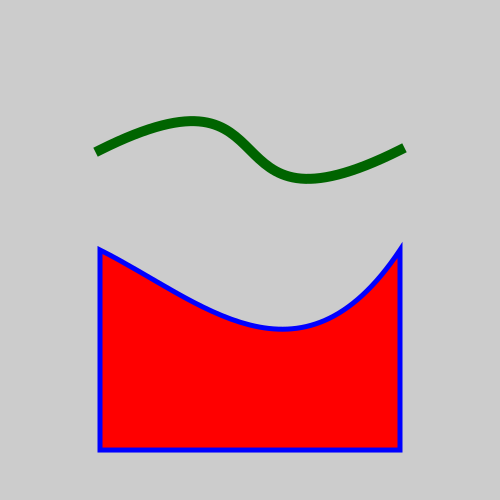# Bezier

Martin McBride, 2020-10-25
Tags geometry bezier
Categories generativepy generative art

The Bezier class draws a bezier curve.

A bezier curve is based on 4 control points, a, b, c and d. The curve starts at point a and ends at point d. The points b and c are called the handles, and they control the shape of the curve.

There is a bezier curves tutorial on PythonInformer that covers this in a lot more detail.

## Bezier class methods

The Bezier class inherits add, fill, stroke, fill_stroke, path, clip and other methods from Shape.

• of_abcd
• of_bcd

### of_abcd

Creates a line based on the 4 control points.

of_abcd(a, b, c, d)

Parameter Type Description
a (number, number) A tuple of two numbers, giving the (x, y) position of the start of the curve.
b (number, number) A tuple of two numbers, giving the (x, y) position of the first handle.
c (number, number) A tuple of two numbers, giving the (x, y) position of the second handle.
d (number, number) A tuple of two numbers, giving the (x, y) position of the end of the curve.

### of_bcd

Creates a line based on the handles and end point only.

of_bcd(b, c, d)

Parameter Type Description
b (number, number) A tuple of two numbers, giving the (x, y) position of the first handle.
c (number, number) A tuple of two numbers, giving the (x, y) position of the second handle.
d (number, number) A tuple of two numbers, giving the (x, y) position of the end of the curve.

This method creates a curve by specifying the handles and end point only. The start point is set to a value of (0, 0).

This is mainly intended for use in composite paths, where lines are joined one after the other. Each line takes its start point as the previous lines end point. See the composite paths tutorial.

## Example

Here is some example code that draws a curve using the class. The full code can be found on github.

from generativepy.drawing import make_image, setup
from generativepy.color import Color
from generativepy.geometry import Bezier, Polygon

'''
Create bezier curve using the geometry module.
'''

def draw(ctx, width, height, frame_no, frame_count):
setup(ctx, width, height, width=5, background=Color(0.8))

# Bezier objects can only be stroked as they do not contain an area.
Bezier(ctx).of_abcd((1, 1.5), (3, 0.5), (2, 2.5), (4, 1.5)).stroke(Color('darkgreen'), 0.1)

# Create a polygon with a bezier side
Polygon(ctx).of_points([(1, 4.5), (1, 2.5), (2, 3, 3, 4, 4, 2.5), (4, 4.5)]).fill(Color('red')).stroke(Color('blue'), 0.05)

make_image("/tmp/geometry-bezier.png", draw, 500, 500)


Here is the image it creates:The top curve is created using the Bezier class.

The bottom shape is created using the Polygon class described here.